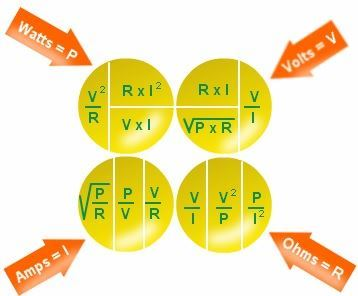# Electricity Formulas

Electricity is the flow of charge in a conductor from anode to cathode. Electricity has varied applications. It acts as a tool to provide power to electrical devices. We can say the flow of charge builds up the current, which we call Electricity. To understand how the Electricity is generated, we need to understand the various basic parameters related to it like the voltage, current, resistance, conductivity and the relation among them.Some of the commonly used Electricity formulae are listed below.

 Quantity Formulas Unit Current I I = Q / t Q = Charge t = time taken Amperes (A) Voltage V V = E / Q or V = W / Q E = Energy, W = Work done Volts (V) Resistance R R = ρl / A ρ = Resistivity, l = length, A = Area or,  R = V / I Ohm (Ω) Power P P = VI Watts (W) Conductivity σ sigma = 1 / ρ Siemens per meter (S/m)

Electricity Formulas are applied in calculating the unknown electrical parameters from the known in electric circuits.

## Solved Examples

Example 1

An electric heater has a potential difference of 220 V and resistance is 70 Ω. Determine the magnitude of the current flowing through it.

Solution:

Given:

Resistance R = 70 Ω

Voltage V = 220 V

The current formula is given by

I = V / R

= 220 / 70

I = 3.1428 A

Example 2

An electrical lamp lights for 4 hours and draws a current of 0.5 A. Calculate the amount of charge flowing through the lamp.

Solution:

Current I = 0.5 A

Time taken t = 4 hours

t= 4 × 3600 = 14400 s,

Charge Q = I × t

= 0.5 ×14400

Q= 7200 C

1. Lochen kumar luitel

Very nicely you are helpful for me coz i understand everything that i know

1. yes even me

2. Mitoo SINGH

I’m a student of class 10 and I was full of doubt about the formulas and I am so happy To understand it by you ,thank you byjus

Thanks for formulas

4. pranjul Bajpai

6. GOVIND SINGH

Very nice sir absolutely byjus is a great website or app

7. Thank you very much byju’s
It helps me a lot

8. Gulshan kumar

You are very helpful for me

9. Prathmesh

Excellent understanding, best quality questions and there solutions . External support of brilliant understanding.

1. It works in board exam 👍

10. Deepjot singh

Thanks I like it

11. Masaud

THANK YOU

12. Deepak

Relly this formula and equation help me to understand the formulas 😃😄😄😀🙂🙂

13. Ngamlem

Good and easy way। Of learning

15. Akhil Vishwakarma

Thank u sooo much for explaining in such a beautiful way.

17. Your electricity formula diagram was very helpful in that it brought back many of the formulas that I have forgotten in my aging years. It is nice to have an easy reference to go to. However, I ask that you review your formula in the upper right hand quadrant where you have V = V/I. Unless I have mistaken the intent, I believe that this should be V = W/I.

18. M Sri vyshnavi

Very nice help thank u for supporting children

19. It really works in board exam 👍👍👍👍👍

20. Maharsh

Very nice I was finding the formula in textbook and now I got all the formula on one website

21. Really helpful specially for the last time formula go through with the units

22. Byjus has always helped me with my studies. Thank u byjus.

23. Sethuram

Thank you

25. Ashutosh kumar

26. Yashvi Jain

This is very easy way to learn and understand this formulas .

27. Swastika

thanks a lot byjus, tomorrow i am having an exam, so I was revising the formulas,and that time i saw that byjus had all formulas in electricity, It helped me a lot ,Thank you.

28. Ayush Raivanshi

Very nice formula for class 10 and very useful

29. Jagdish

Thanks you have helped me a lot

30. Archita Munshi

Thank you BYJUS, your notes are absolutely great.

31. It literally helped

32. Natasha

Thank you sir/mam for the formulas and examples, it helped me a lot.